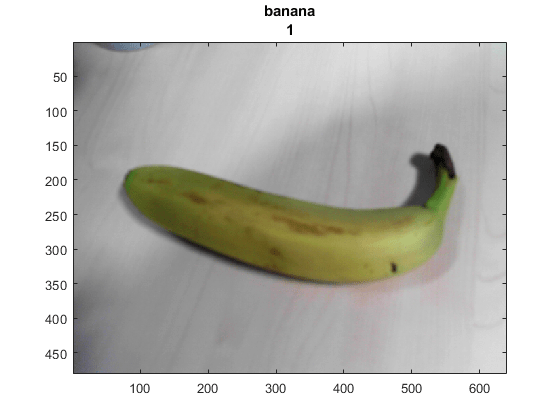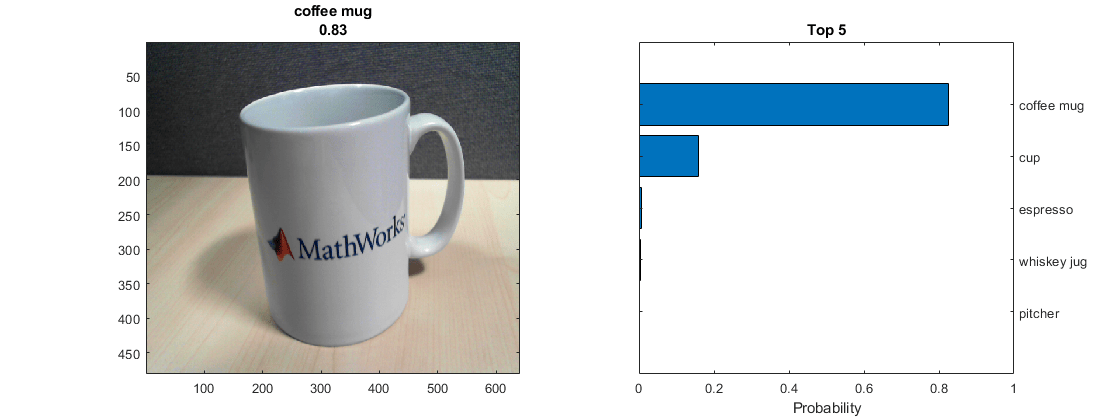# 使用深度学习对网络摄像头图像进行分类

### 加载相机和预训练网络

```camera = webcam; net = googlenet; ```

### 对相机快照进行分类

```inputSize = net.Layers(1).InputSize(1:2) ```
```inputSize = 224 224 ```

```figure im = snapshot(camera); image(im) im = imresize(im,inputSize); [label,score] = classify(net,im); title({char(label),num2str(max(score),2)}); ```### 连续对相机图像进行分类

```h = figure; while ishandle(h) im = snapshot(camera); image(im) im = imresize(im,inputSize); [label,score] = classify(net,im); title({char(label), num2str(max(score),2)}); drawnow end ```

### 显示排名靠前的预测值

```h = figure; h.Position(3) = 2*h.Position(3); ax1 = subplot(1,2,1); ax2 = subplot(1,2,2); ```

```im = snapshot(camera); image(ax1,im) im = imresize(im,inputSize); [label,score] = classify(net,im); title(ax1,{char(label),num2str(max(score),2)}); ```

```[~,idx] = sort(score,'descend'); idx = idx(5:-1:1); classes = net.Layers(end).Classes; classNamesTop = string(classes(idx)); scoreTop = score(idx); ```

```barh(ax2,scoreTop) xlim(ax2,[0 1]) title(ax2,'Top 5') xlabel(ax2,'Probability') yticklabels(ax2,classNamesTop) ax2.YAxisLocation = 'right'; ```### 连续分类图像并显示排名靠前的预测值

```h = figure; h.Position(3) = 2*h.Position(3); ax1 = subplot(1,2,1); ax2 = subplot(1,2,2); ax2.PositionConstraint = 'innerposition'; ```

```while ishandle(h) % Display and classify the image im = snapshot(camera); image(ax1,im) im = imresize(im,inputSize); [label,score] = classify(net,im); title(ax1,{char(label),num2str(max(score),2)}); % Select the top five predictions [~,idx] = sort(score,'descend'); idx = idx(5:-1:1); scoreTop = score(idx); classNamesTop = string(classes(idx)); % Plot the histogram barh(ax2,scoreTop) title(ax2,'Top 5') xlabel(ax2,'Probability') xlim(ax2,[0 1]) yticklabels(ax2,classNamesTop) ax2.YAxisLocation = 'right'; drawnow end ```